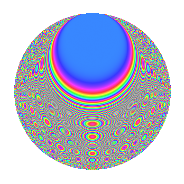# Properties

 Label 162.2.gLevel $162$ Weight $2$ Character orbit 162.g Rep. character $\chi_{162}(7,\cdot)$ Character field $\Q(\zeta_{27})$ Dimension $162$ Newform subspaces $2$ Sturm bound $54$ Trace bound $1$

# Related objects

## Defining parameters

 Level: $$N$$ $$=$$ $$162 = 2 \cdot 3^{4}$$ Weight: $$k$$ $$=$$ $$2$$ Character orbit: $$[\chi]$$ $$=$$ 162.g (of order $$27$$ and degree $$18$$) Character conductor: $$\operatorname{cond}(\chi)$$ $$=$$ $$81$$ Character field: $$\Q(\zeta_{27})$$ Newform subspaces: $$2$$ Sturm bound: $$54$$ Trace bound: $$1$$ Distinguishing $$T_p$$: $$5$$

## Dimensions

The following table gives the dimensions of various subspaces of $$M_{2}(162, [\chi])$$.

Total New Old
Modular forms 522 162 360
Cusp forms 450 162 288
Eisenstein series 72 0 72

## Trace form

 $$162q + O(q^{10})$$ $$162q - 9q^{18} - 18q^{20} - 54q^{21} - 54q^{23} - 54q^{26} - 54q^{27} - 54q^{29} - 54q^{30} - 54q^{33} - 54q^{35} - 18q^{36} - 9q^{38} - 18q^{41} - 54q^{45} - 54q^{47} - 63q^{51} - 54q^{53} - 54q^{57} - 63q^{59} - 54q^{63} + 18q^{65} + 72q^{66} - 54q^{67} + 18q^{68} + 126q^{69} - 54q^{70} + 144q^{71} + 72q^{72} + 144q^{74} + 180q^{75} - 27q^{76} + 288q^{77} + 144q^{78} - 108q^{79} + 36q^{80} + 144q^{81} + 144q^{83} + 36q^{84} - 108q^{85} + 144q^{86} + 288q^{87} - 27q^{88} + 171q^{89} + 144q^{90} + 72q^{92} + 126q^{93} - 54q^{94} + 90q^{95} + 18q^{96} - 54q^{97} + 72q^{98} + 18q^{99} + O(q^{100})$$

## Decomposition of $$S_{2}^{\mathrm{new}}(162, [\chi])$$ into newform subspaces

Label Dim. $$A$$ Field CM Traces $q$-expansion
$$a_2$$ $$a_3$$ $$a_5$$ $$a_7$$
162.2.g.a $$72$$ $$1.294$$ None $$0$$ $$0$$ $$0$$ $$0$$
162.2.g.b $$90$$ $$1.294$$ None $$0$$ $$0$$ $$0$$ $$0$$

## Decomposition of $$S_{2}^{\mathrm{old}}(162, [\chi])$$ into lower level spaces

$$S_{2}^{\mathrm{old}}(162, [\chi]) \cong$$ $$S_{2}^{\mathrm{new}}(81, [\chi])$$$$^{\oplus 2}$$